# Evaluation

## Comparison of CFD Calculations with Experiments

In this section we first present major results of RANS computations of the considered flow performed with different turbulence models [‌7] and their comparison with the experimental data (next subsection) Then, in the following sub-section, results are presented of the scale-resolving simulations (enhanced RANS-LES methods [‌8], [‌9] and WRLES [‌6]). This sub-section begins with a comparison of flow visualizations from different simulations, which visually display turbulence resolving capabilities of the approaches used. Then, a comparison with the experimental data is shown for the main body of these simulations.

### RANS Calculations

The 2DWMH flow has been computed and discussed in numerous RANS studies both by individual researches and in the framework of different collaborative projects and workshops. So below we present only a concise outline of major findings of these studies based on quite representative information on performance of different RANS models available at https://turbmodels.larc.nasa.gov/nasahump_val.html [‌7]. The models (see Table 5) include: four linear eddy viscosity models (one-equation model of Spalart & Allmaras (SA model) [‌31], this model with the Rotation-Curvature correction (SACC) [‌32], the two-equation k-ω Shear Stress Transport of Menter (SST) [‌33]) and the two-equation k-kL model of Menter & Egorov and Abdol-Hamid (k-kL-MEAH2015 [‌34]) and one differential Reynolds Stress Model (RSM), namely the SSG/LLR-RSM-w2012 model [‌35] which “blends” the Speziale-Sarkar-Gatski (SSG) model [‌36] in the near wall flow region and Launder-Reece-Rodi (LRR) model [‌37] in the outer region.

Figures 89 show plots of distributions of the pressure and friction coefficients over the hump predicted by different linear eddy viscosity models (Fig. 8) and by the RSM (Fig. 9) with the use of the two NASA codes (structured code CFL3D and unstructured code UNS3D) together with the corresponding experimental data. The figures show that in terms of agreement with the data, none of the models ensures accurate prediction of the pressure and friction distributions and that all of them considerably over-predict the reattachment location and the length of the separation bubble. Other than that, the k-kL-MEAH15 model exhibits a very poor prediction of Cf. The shortest bubble is predicted by the RSM, but it still remains roughly 25% longer than in the experiment. Moreover, as noted in [‌38], the SSG/LLR-RSM-w2012 predicts an unnatural back bending of the streamline near reattachment (see Fig. 10).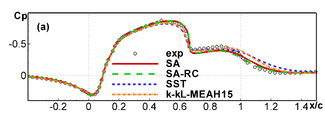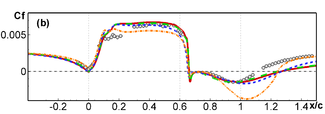Figure 8: Comparison with experiment of streamwise distributions of the pressure (a) and friction (b)coefficients predicted by linear eddy-viscosity turbulence models [‌7]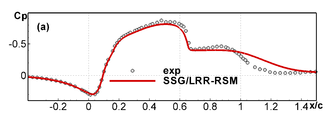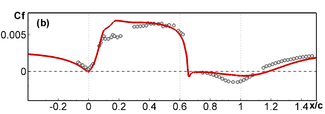Figure 9: Same as in Fig. 8 for RSM model SSG/LLR-RSM-w2012 [‌7]

Table 5: Prediction of separation and reattachment locations by CFL3D and UNS3D codes with different RANS turbulence models [‌7]
Model xsep / c xreattach / c (xreattach - xsep) / c
SA  0.661 1.26 0.599
SARC  0.661 1.23 0.569
SST  0.654 1.26 0.606
k-kL-MEAH2015  0.658 1.24 0.582
SSG/LLR-RSM-w2012  0.653 1.18 0.527
Experiment [‌1], [‌2] 0.665 1.10 0.435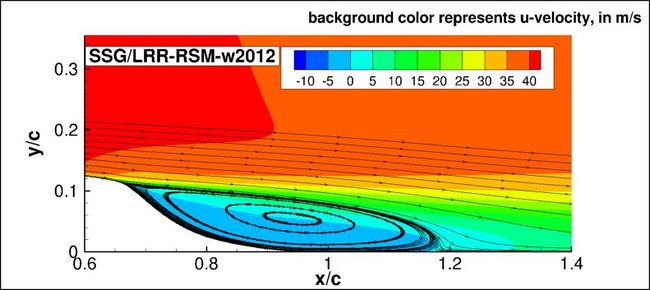Figure 10: Streamwise velocity contours and streamlines predicted by SSG/LRR-RSM-w2012 RANS model [‌7]

Note that the over-prediction of the length of the separation bubble by RANS models is commonly associated with a dramatic underestimation of the peak of the Reynolds shear stress in the separated shear layer, which is typical of these models. The latter trend is clearly seen in Fig. 11.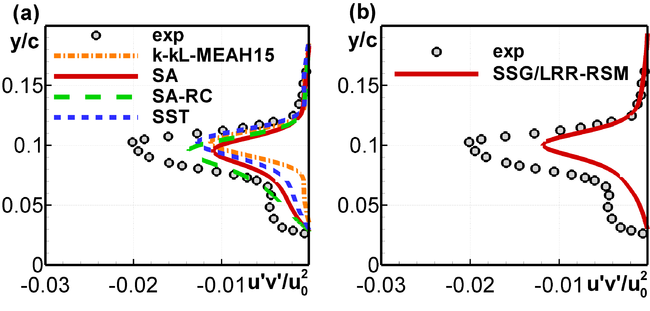Figure 11: Reynolds shear stress profiles predicted by linear eddy-viscosity (a) and RS (b) RANS models atx/c = 0.8 [‌11]

### RANS-LES Hybrids and Wall Resolved LES

#### Sensitivity Tests

As mentioned above, the hybrid RANS-LES simulations presented and briefly analyzed in this sub-section were carried out in the framework of the collaborative EU project Go4Hybrid with the use of “mandatory” computational problem setup and grid outlined in the previous section. Although both setup and grid had been carefully designed based on results of previous studies of the 2DWMH flow, no sensitivity studies aimed at evaluation of the effect of computational uncertainties had been performed. In contrast to this, within the WRLES investigation [‌6], such studies were carried out. They included evaluation of the following effects:

• SGS model (static SGS model of Vreman vs. no-model simulation (ILES)).
• Size of the domain in the uniform (spanwise) direction (0.2c vs. 0.4c).
• Grid-refinement (850 million vs. 420 million grid points in the wide domain simulations).
• Shape of incoming upstream boundary layer (velocity and Reynolds stress profiles from the DNS of the zero pressure gradient turbulent boundary layer at Reθ = 5000, which matches the experimental skin-friction, vs. the mean velocity profile available from RANS, which matches the experimental velocity profile – see Fig. 4).
• Top wall contour (original, shown in Fig. 5 vs. the contour with 50% increased displacement).
• Mach number (0.2 vs. the experimental value of 0.1) and tunnel back pressure (pb / pref = 0.998 vs. 1.001).

Results of all these studies are discussed in detail in [‌6], and so we do not present them here. Note only that, although all the effects listed above result in some subtle alterations of the obtained solutions, they do not cause any significant change of the major predicted flow characteristics. For example, the minimum and maximum values of the coordinate of the reattachment point xreattach/c, whose prediction in the considered flow is the most challenging, in all these simulations varies from ≈ 1.059 up to ≈ 1.091 (the experimental value is ≈ 1.1).

#### Flow Visualizations

Figures 1213 show flow visualizations from simulations carried out with TAU and NTS codes within different hybrid RANS-LES approaches. Analysis of these figures allows drawing the following conclusions.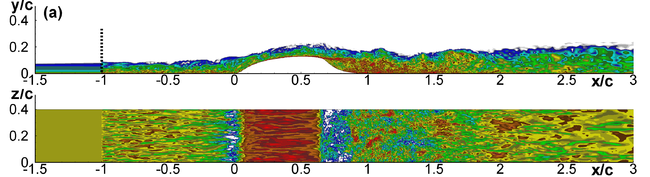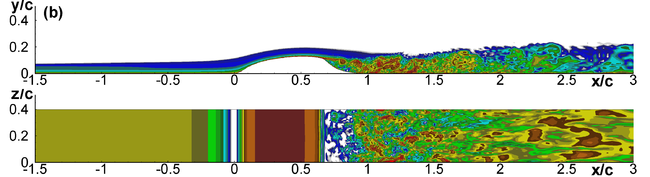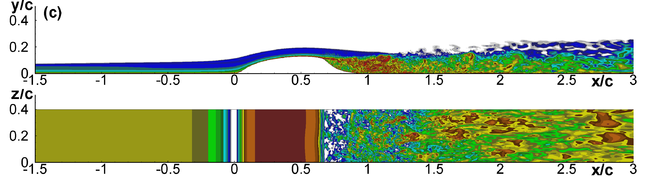Figure 12: Comparison of snapshots of vorticity magnitude in XY-plane and on the hump wall from simulations performed with NTS code. (a): zonal RANS - IDDES with interface at x/c = -1.0;(b): non-zonal DDES with Δmax; (c): non-zonal DDES with ΔSLA.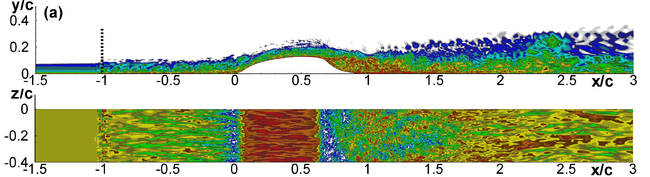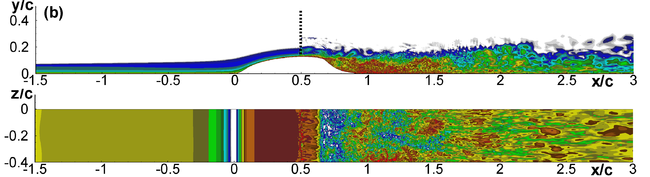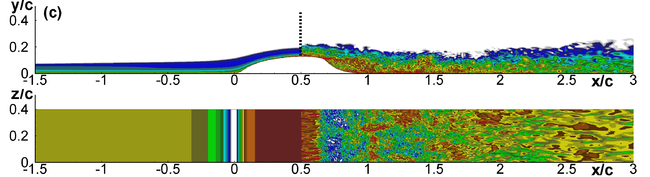Figure 13: Same, as in Fig. 12, from zonal RANS-IDDES performed with TAU code (a), (b) and NTS code (c).(a): interface at x/c = -1.0; (b) and (c): at x/c = 0.5

First, the zonal RANS-IDDES combined with both STG [‌13] and SEM [‌14],  [‌15] ensure a rapid formation of developed 3D turbulence downstream of the RANS-IDDES interface independently of its location (in the zero pressure gradient boundary layer at x/c = -1.0 or right upstream the separation at x/c = 0.5). However, turbulent structures in the close vicinity of the interface predicted in the TAU and NTS simulations look somewhat different (compare frames (a) in Figs. 12 and 13 and frames (b) and (c) in Fig. 13). This is not surprising considering a significant difference of the turbulence generators used in the codes. At the same time, further downstream no tangible qualitative difference of the resolved turbulence is observed. Hence, judging by the flow visualizations, both generators ensure rather short relaxation lengths within the two codes. Second, all the zonal simulations indicate a resolution of fine turbulent structures compatible with the cells sizes in the “focus” region located downstream of the separation point, thus suggesting that the unstructured TAU code using the LD2 scheme provides low numerical dissipation comparable with that of the higher-order structured NTS code. Finally, a comparison of the flow visualizations from the zonal RANS-IDDES (Fig. 12 (a)) and global DDES with the shear layer adapted length scale ΔSLA (Fig. 12 (c)) shows that downstream of the separation both approaches return similar solutions and, in particular, ensure a rapid break-up of the separated shear layer and transition to developed 3D turbulence. In contrast to this, the original DDES with Δmax (Fig. 12 (b)) reveals a considerable delay of formation of the resolved 3D turbulent structures in the separated shear layer. This supports the high efficiency of the ΔSLA definition of the subgrid length scale in terms of the mitigation of the grey area.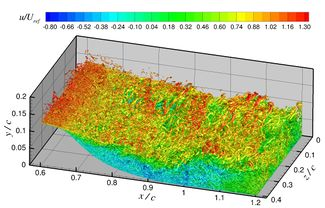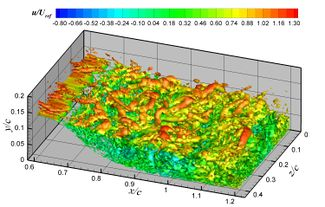Figure 14: Instantaneous iso-surface of Q-criterion coloured by streamwise velocity: (a) WRLES [‌6], Q = 150U0/c; (b): zonal RANS-IDDES with interface at x/c = -1.0 from simulation performed with the NTS code [‌8], [‌9] Q = 20U0/c

The last flow visualizations presented in Fig. 14 give an idea about the considerable difference of the size of turbulent structures resolved by the WRLES [‌6] and by hybrid RANS-IDDES [‌8], [‌9] in the focus region of the flow.

#### Comparison with Experiment

Figures 15 — 19 compare distributions of the mean pressure and friction coefficients over the hump wall predicted by all the considered simulations.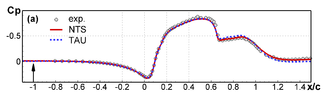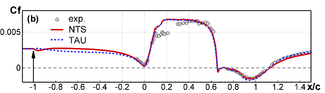Figure 15: Streamwise distributions of the pressure (a) and friction (b) coefficients from zonal RANS-IDDES simulations performed with the use of NTS and TAU codes with interface at x/c = -1.0.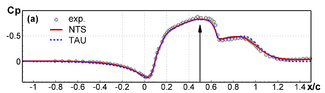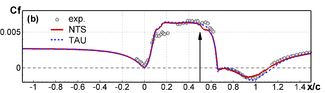Figure 16: Same, as in Fig. 15, from zonal RANS-IDDES with interface at x/c = 0.5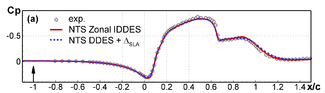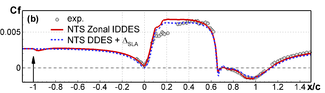Figure 17: Same, as in Fig. 15, from zonal RANS-IDDES with interface at x/c = -1.0 and from non-zonal DDES with ΔSLA computed with the use of NTS code.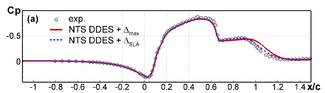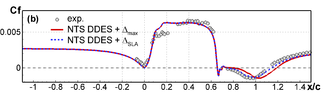Figure 18: Same, as in Fig. 15, from non-zonal DDES with ΔSLA and Δmax computed with the use of NTS code.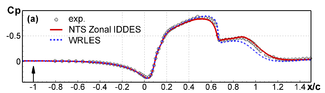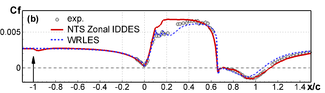Figure 19: Same, as in Fig. 15, from WRLES [‌6] and from zonal RANS-IDDES at x/c = -1.0 computed with the use of NTS code.

In line with the expectations based on the flow visualizations, predictions of all the hybrid simulations (see Figs. 15 – 18) turn out to be close to each other and, in contrast to the RANS predictions (Figs. 8, 9), they all agree well with the experiment. Other than that, Fig. 18 demonstrates that the non-zonal DDES approach combined with the length scale ΔSLA performs considerably better than the standard DDES (with Δmax) and is quite competitive with the zonal RANS-IDDES. Its skin-friction distribution is naturally free from local disturbances due to injection of synthetic-turbulence at the RANS-IDDES interface in the zonal simulations but, on the other hand, it yields a slightly over-predicted separation zone, which is a “remainder” of the grey area issue.

Figure 19 compares pressure and friction distributions computed with the use of the zonal RANS-IDDES with the interface located at x/c = -1.0 and with the use of WRLES [‌6]. A remarkable feature of the latter is its capability of capturing a plateau of the friction coefficient curve observed in the experiment at 0.3 ≥ x/c ≥ 0.1, i.e., in the region of the flow acceleration driven by a strong favorable pressure gradient which, as argued in [‌6], results in a tendency to relaminarization of the boundary layer [‌6]. All the hybrid models almost completely miss this effect and predict only a minor “dent” in the friction distribution (see Figs. 15 – 18. On the other hand, the WRLES predicts the reattachment point a bit earlier than in the experiment and in this respect is slightly inferior to the zonal RANS-IDDES with the interface located at x/c = -1.0 (see Table 6).

Table 6: Prediction of separation and reattachment locations by different codes and modelling approaches
Approach & Code RANS-LES interface Separation point, x / c Reattachment point, x / c (xreattach - xsep) / c
Zonal RANS-IDDES combined with SEM; TAU code x/c = 0.5
x/c = -1.0
0.655
0.656
1.152
1.114
0.497
0.458
Zonal RANS-IDDES combined with STG; NTS code x/c = 0.5
x/c = -1.0
0.658
0.656
1.12
1.131
0.462
0.475
Non-zonal DDES combined with ΔSLA; NTS code 0.656 1.143 0.487
Standard (with Δmax) non-zonal DDES; NTS code
Wall-Resolved ILES; code  0.661 1.064 0.403
Experiment 0.665 1.11 0.445

Figures 2021 present comparisons of the profiles of the mean streamwise velocity (Fig. 20) and shear Reynolds stress (Fig. 21) at different flow cross-sections predicted by all the considered simulations and measured in the experiment.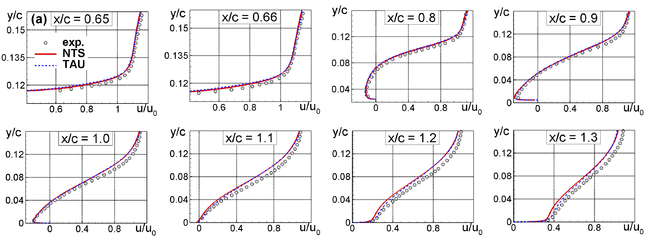(a): Zonal RANS – IDDES with interface located at x/c = -1.0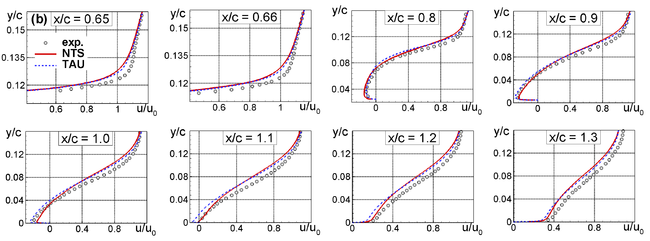(b): Zonal RANS – IDDES with interface located at x/c = 0.5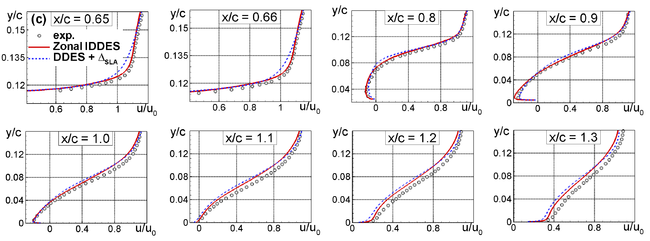(c): Non-zonal DDES with ΔSLA subgrid scale and zonal RANS – IDDES with interface located at x/c = -1.0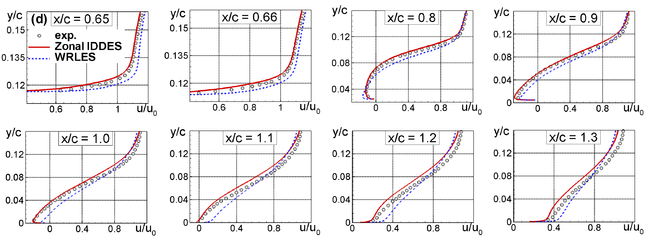(d): zonal RANS – IDDES with interface located at x/c = -1.0 and Wall Resolved ILES.
Figure 20: Comparison of predicted and measured streamwise velocity profiles at different flow cross-sections

General observations based on analysis of Fig. 20 are similar to those based on the comparison of the computed and measured pressure and friction distributions. However the velocity profiles predicted by the zonal RANS-IDDES methods at 0.65 ≤ x/c ≤ 0.9 turn out to be somewhat more sensitive to the location of the RANS-IDDES interface than the Cf and, especially CP distributions. Other than that, in the simulations with the interface located at x/c = 0.5 the difference between the TAU and NTS codes predictions becomes a bit larger than with the interface at x/c = -1.0, which is best seen by comparing the profiles at 1.0 ≤ x/c ≤ 1.2 in Fig. 20 (a) and Fig. 20 (b). In general, placing the RANS-IDDES interface farther upstream of the hump (at x/c = -1.0) ensures somewhat better agreement with the experiment than placing it at x/c = 0.5. In particular, the two profiles just around the separation point (x/c = 0.65 and 0.66) are best predicted with the far-upstream interface (Fig. 20(a)), whereas the downstream interface (Fig. 20(b)) and the non-zonal DDES (Fig. 20(c)) yield a slightly too large momentum loss in this critical region. Moreover, all the simulations tend to over-predict the near-wall momentum loss around reattachment and recovery, although to a different degree. In line with the mean surface data in (Fig. 17), the non-zonal DDES with ΔSLA (Fig. 20(c)) predicts slightly larger deviations from the experiment than the zonal computations at all considered cross-sections.

Finally, as seen in (Fig. 20(d)), a deviation from the experiment of the velocity profiles predicted by the WRLES is opposite and somewhat larger compared to that of the profiles predicted by the RANS-IDDES methods, which is consistent with the corresponding predictions of the skin-friction distributions.(a): Zonal RANS – IDDES with interface located at x/c = -1.0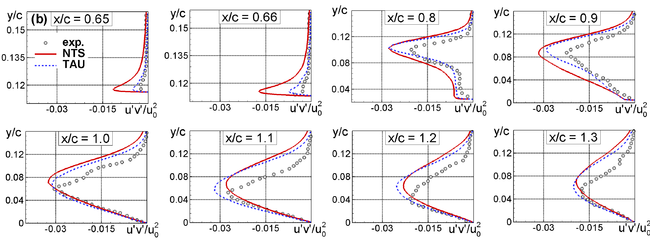(b): Zonal RANS – IDDES with interface located at x/c = 0.5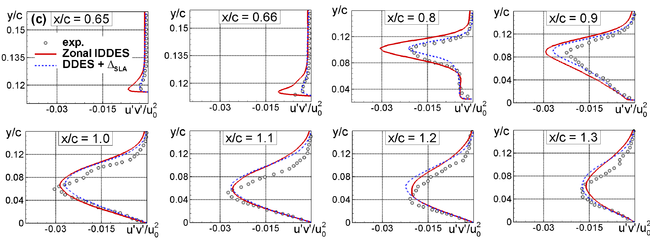(c): Non-zonal DDES with ΔSLA subgrid scale and zonal RANS – IDDES with interface located at x/c = -1.0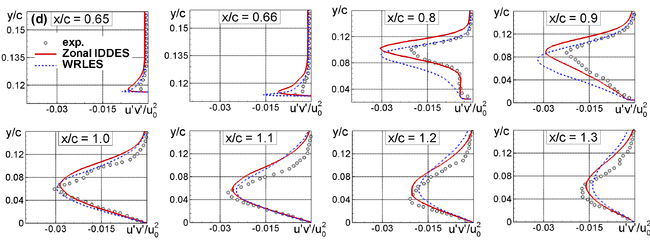(d): zonal RANS – IDDES with interface located at x/c = -1.0 and Wall Resolved ILES.
Figure 21: Comparison of predicted and measured Reynolds shear stress profiles at different flow cross-sections

Figure 21 compares Reynolds shear stress profiles predicted by all the considered simulations and the measured Reynolds shear stress in the same flow cross-sections as the velocity profiles in Fig. 20.

As seen in Fig. 21(a), for the interface located at x/c = -1.0, both NTS and TAU codes overestimate the experimental peak values of the stress within the recirculation bubble (0.65 ≤ x/c ≤ 1.0), the difference being larger in the simulations performed with the TAU code. This trend is opposite to that observed in the RANS computations (see Fig. 11) Further downstream (x/c = 1.0) the discrepancy between the simulations and the experiment, as well as between the two simulations decreases. With the interface location at x/c = 0.5 (Fig. 21(b)), the difference between the NTS and TAU predictions becomes smaller, except for the very beginning of the separated shear layer (x/c = 0.65 and 0.66), where the NTS code strongly overestimates the peak stress values (this is probably explained by injecting the synthetic turbulence too close to the separation). However, due to the strong sudden pressure rise right upstream of the separation, its location is virtually not affected by this discrepancy (see Table 6). Still, as could be expected based on the analyses of predicted velocity profiles and pressure and friction distributions, the agreement of the simulations with the experiment becomes somewhat worse compared to the simulation with the interface at x/c = -1.0.

Figure 21(c) compares performance of the zonal RANS-IDDES approach and non-zonal DDES combined with the ΔSLA definition of the subgrid length scale. It shows that in terms of agreement with the measured stresses the non-zonal approach appears to be the best one. This suggests that the use of the shear-layer adapted definition of the subgrid length scale is an efficient grey area mitigation tool but, on the other hand, shows once again that an accurate prediction of the shear stress alone does not guarantee overall accuracy of simulations.

Finally, Fig. 21(d) compares shear stress profiles predicted by the zonal RANS-IDDES with the interface located at x/c = -1.0 and by the Wall Resolved ILES. The comparison shows that a major difference between the two approaches is observed in the initial part of the recirculation zone where the location of the peak shear stress predicted by the ILES is shifted to the wall compared to that predicted by the zonal approach and observed in the experiment. In the region downstream of the reattachment predictions of the both approaches are very close to each other and fairly well agree with the data.

To summarize, taking into account the uncertainties with respect to both the measurements and the simplified (quasi-2D with approximate account for the blockage effect of the side plates) simulations setup, all the observed discrepancies of scale-resolving approaches to turbulence representation with the data may be characterized as insignificant, especially if to compare them with the corresponding discrepancies of the RANS predictions (see RANS Calculations). Hence, a general conclusion based on the presented results of the simulations is that the zonal RANS- IDDES combined with synthetic turbulence generators, non-zonal DDES combined with shear layer adapted subgrid length scale, and WRLES are all quite capable of fairly accurate predicting the considered test case.

1. Similar comparisons of the lateral mean velocity component and normal Reynolds stresses are not presented here but can be made based on the data available at https://turbmodels.larc.nasa.gov/Other_LES_Data/nasa_hump_uzun_2017.html
2. This suggests that underestimation of the maximum shear stress in the separated shear layer (see RANS Calculations subsection) is not the only reason of the over-prediction of the coordinate of the separation point by the RANS models.

Contributed by: E. Guseva, M. Strelets — Peter the Great St. Petersburg Polytechnic University (SPbPU)